# Solve equation

solve equation:

$\left[\left({a}^{2}\right)+3{\right]}^{1\mathrm{/}2}+\left[\left({a}^{2}\right)-3{\right]}^{1\mathrm{/}2}=5$

Correct result:

a1 =  0
a2 =  0

#### Solution:

${a}_{1}=\sqrt{661}\mathrm{/}10\doteq 2.571=0$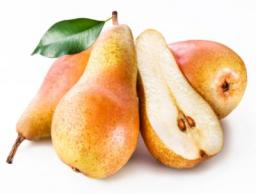We would be pleased if you find an error in the word problem, spelling mistakes, or inaccuracies and send it to us. Thank you!Tips to related online calculators
Looking for help with calculating roots of a quadratic equation?
Do you have a linear equation or system of equations and looking for its solution? Or do you have quadratic equation?

## Next similar math problems:

• Variation equation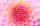Solve combinatorics equation: V(2, x+8)=72
• DiscriminantDetermine the discriminant of the equation: ?
• CalculationHow much is sum of square root of six and the square root of 225?
• EquationEquation ? has one root x1 = 8. Determine the coefficient b and the second root x2.
• Reciprocal equation 2Solve this equation: x + 5/x - 6 = 4/11
• Reciprocal value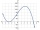How do I calculate a number x that is 9 greater than its reciprocal (1/x)?
• VariableFind variable P: PP plus P x P plus P = 160
• BirthdaysIn the classroom, students always give candy to their classmates on their birthdays. The birthday person always gives each one candy, and he does not give himself. A total of 650 candies were distributed in the class per year. How many students are in the
• ProductThe product of two consecutive odd numbers is 8463. What are this numbers?
• Solve 3Solve quadratic equation: (6n+1) (4n-1) = 3n2Find the roots of the quadratic equation: 3x2-4x + (-4) = 0.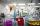The chemist wanted to check the content of water of crystallization of chromic potassium alum K2SO4 * Cr2 (SO4) 3 * 24 H2O, which was a long time in the laboratory. From 96.8 g of K2SO4 * Cr2 (SO4) 3 * 24 H2O prepared 979 cm3 solution of base.Determine the quadratic equation absolute coefficient q, that the equation has a real double root and the root x calculate: ?If a2-3a+1=0, find (i)a2+1/a2 (ii) a3+1/a3If the square root of 3m2 +22 and -x = 0, and x=7, what is m?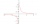Find value of unknown x in equation: x+3/x+1=5 (problem finding x)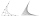In the equation 2x ^ 2 + bx-9 = 0 is one root x1 = -3/2. Determine the second root and the coefficient b.